SYLLABUS  Previous: 5.3 Particle orbits  Up: 5 MONTE-CARLO METHOD  Next: 5.5 When should you

## 5.4 A scheme for the advection diffusion equation

Slide : [ scheme - Box-Mueller || VIDEO login]

Let us use now the Feynman-Kac theorem and evolve a large number of stochastic particle orbits to approximate the advection diffusion equation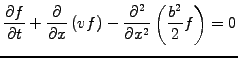(1)

The rules of the Itô calculus require the time discretization to be explicit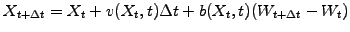(2)

Note that it is in fact possible to construct implicit discretizations of a stochastic differential equation , but the construction it not as simple as it is for ordinary equations (5.3#eq.3). Since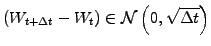, the Wiener process can be written explicitly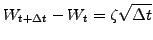(3)

where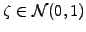are normally distributed random numbers. The central limit theorem states that any large sum involving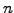random numbers equally distributed with zero mean and unit variance will eventually converge to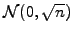. Any such random number could therefore be used for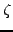provided that the number of time steps used to approximate the differential is large enough; choosinghowever leads to the fastest convergence.

A numerical Monte Carlo scheme for the advection-diffusion equation is now constructed, using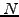particles to sample the possible outcomes of the entire ensemble of orbits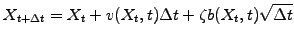(4)

This is simply be implemented as
      for(int j = 0; j < numberOfParticles; j++){
particlePosition[j] += velocity * timeStep +
random.nextGaussian() *
Math.sqrt(2 * diffusCo * timeStep);
// Insert here your periodic boundary conditions (exercise 5.03)
}

and can be tested in the JBONE applet below

 Numerical experiments: Monte Carlo scheme for advection-diffusion Compare the solution obtained with 128 steps of duration TimeStep=0.5 with the one achieved with a single step of duration TimeStep=64; which is faster and which is more precise? Observe how the periodic boundary conditions have been implemented so that particles can take steps that are larger than the domain size (exercise 5.03).

JAVA is one of the few programming languages that has a normal pseudo random numbers generator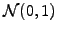. Other programming languages generally provide only uniform pseudo random numbers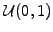and the Box Müller method can then be used to transfrom them intowith the algorithm:

• Construct two uniformly distributed random numbers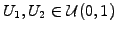.
• Then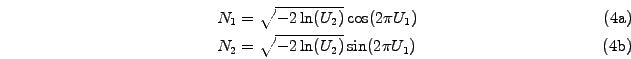are two independent pseudo random numbers in.
The rest of the implementation is equivalent if one remembers the definition of the command x+=dx as being equivalent to x=x+dx.

SYLLABUS  Previous: 5.3 Particle orbits  Up: 5 MONTE-CARLO METHOD  Next: 5.5 When should you